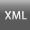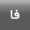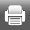Volume 4, Issue 2 (9-2016)jgit 2016, 4(2): 47-64 Back to browse issues page
Determining regularization parameter in high resolution images georefrencing with Rational Functions
Amir Zafari *, Alireza Amiri simkooei, Mehdi Momeni
Isfahan University
Abstract:   (3030 Views)

High-resolution satellite images are extensively used in different fields. Geo-referencing process, as innate part of extraction of topographic terrains through these images, has been studied in many researches. In geo-referencing of satellite images, different models can be used, but rational functions are the most suitable options. Determining co-efficiency of rational functions is ill-condition problem, so to solve this problem Tikhonov regularization method has been used. In such regularization method, regularization selection parameter is very important. In present study, this parameter was calculated through two methods including: minimizing root mean square of errors (RMSE) and the L-curve for determining co-efficiency of rational functions. Then these two methods have been used in least standard squares of parametric model. Also combined model has been used to determine co-efficiency of rational functions in geo-referencing process. These calculations have been done for two different control-points groups with various numbers and accuracies. Using these two models (parametric and combined), regularization parameter has been calculated through L-curve and root mean square of error methods by 55 points. The results show that the root mean square errors and L-curve methods in parametric model led to accuracy of 4.45 and 5.40 pixels, respectively. Also in the combined model, root mean square errors and L-curve methods showed accuracy of 3.42 and 5.10 pixels, respectively. Above calculations were repeated with 120 points. This time, results show approximately same accuracies for both root mean square errors and L-curve methods.

Keywords: High-Resolution Satellite Images, Rational Function, Ill-ondition Problem, regularization, L-curve.Rights and permissionsThis work is licensed under a Creative Commons Attribution-NonCommercial 4.0 International License.